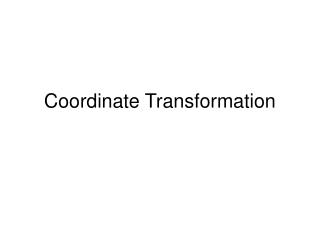DownloadDownload PresentationCoordinate Transformation

# Coordinate Transformation

Download Presentation## Coordinate Transformation

- - - - - - - - - - - - - - - - - - - - - - - - - - - E N D - - - - - - - - - - - - - - - - - - - - - - - - - - -
##### Presentation Transcript

1. Coordinate Transformation

2. How to transform coordinates from one system to another.In this situation we have earth coordinates on the left and digitizer coordinates on the right. So we are transforming between locations on the globe (i.e. geographic coordinates) and the coordinate system of the digitizing table (i.e. millimeters).

3. What we want • We would like to click on the digitizing “puck” and get a UTM coordinate placed in our GIS. • In order to do this we need to calculate the “transformation parameters” between the UTM coordinate system and the coordinate system of the Digitizing Tablet. • To align these systems we go through a translation, a scaling and a rotation.

4. x y x’ y’ Translation x’ = Tx + x y’ = Ty + y N Tx Ty E

5. y’ Scaling x’’ = SN” * x’ y’’ = SE” * y’ N y’’ x’’ x’ E

6. xa’’ cos q Rotation N = xa’’sinq + ya’’cosq E = xa’’ cosq – ya’’sinq N Ea,Na y’’ y’’a x’’a ya’’ cos q ya’’ sin q x’’ q xa’’ sin q E

7. The transformation equations • The final transformation equations are: • N = a1 + a2x + a3y • E = b1 + b2x + b3y • Where: the a1 and b1 contain the translation parameters and a2,a3,b2, andb3 contain the scale and rotation parameters. • a1, a2,a3,b1,b2, andb3 are the unknown coefficients that need to be solved in order to transform from the (x,y) coordinate system to the (N,E) coordinate system.

8. Solving for the Unknown coefficientsTo solve for the unknowns we need to select a least four coordinates that can be physically identified in both of the coordinate systems. Road intersections are often used as well as geographic features such as the tip of a peninsula. (N1,E1) (N2,E2) (x2,y2) (x1,y1) (x3,y3) (N3,E3) (x4,y4) (N4,E4)

9. Solving for the Unknown coefficients (cont) • With four points we can write 8 equations one for each x and y coordinate. These equations are solved simultaneously to yield the 6 coefficients. By plugging these coefficients back into the equations we can solver for the residuals (vy, vx) which gives us the accuracy of our transformation. • N1 = a1 + a2x1 + a3y1 N1 = a1 + a2x1 + a3y1 + vy1 • E1 = b1 + b2x1 + b3y1 E1 = b1 + b2x1 + b3y1 + vx1 • N2 = a1 + a2x2 + a3y2 N2 = a1 + a2x2 + a3y2 + vy2 • E2 = b1 + b2x2 + b3y2 Least Squares solution E2 = b1 + b2x2 + b3y2 + vx2 • N3 = a1 + a2x3 + a3y3 N3 = a1 + a2x3 + a3y3 + vy3 • E3 = b1 + b2x3 + b3y3 Plug in coefficients to solve for residuals E3 = b1 + b2x3 + b3y3 + vx3 • N4 = a1 + a2x4 + a3y4 N4 = a1 + a2x4 + a3y4 + vy4 • E4 = b1 + b2x4 + b3y4 E4 = b1 + b2x4 + b3y4 + vx4

10. How it works.Now we can transform our digitizer coordinates into the coordinate system of the map. b1 = 488,524.790 b2 = 150.0567 b3 = 234.0678 a1 = 4,831,480.600 a2 = 560.546 a3 = 465.347 X = 23.4567 Y = 33.9875 • N1 = a1 + a2(23.4567) + a3(33.9875) • E1 = b1 + b2(23.4567) + b3 (33.9875) • N1 = 4,831,480.60 + 560.546(23.4567) + • 465.347(33.9875) • E1 = 488,524.79 + 150.0567(23.4567) + • 234.0678 (33.9875) GIS N1 = 4,871,656. E1 = 500,000.